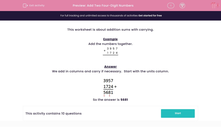# Subtracting From Positive and Negative Numbers

In this worksheet, students subtract numbers from positive and negative numbers.Key stage:  KS 3

Curriculum topic:   Number

Curriculum subtopic:   Use Four Operations for All Numbers

Difficulty level:#### Worksheet Overview

This worksheet is about subtracting something from positive and negative numbers.

Examples

 420 − 421 = -1

−160 − 160 =
 −320

Think of a number line if it helps.  When you subtract, you move to the left to make a number "more negative".

### What is EdPlace?

We're your National Curriculum aligned online education content provider helping each child succeed in English, maths and science from year 1 to GCSE. With an EdPlace account you’ll be able to track and measure progress, helping each child achieve their best. We build confidence and attainment by personalising each child’s learning at a level that suits them.

Get started